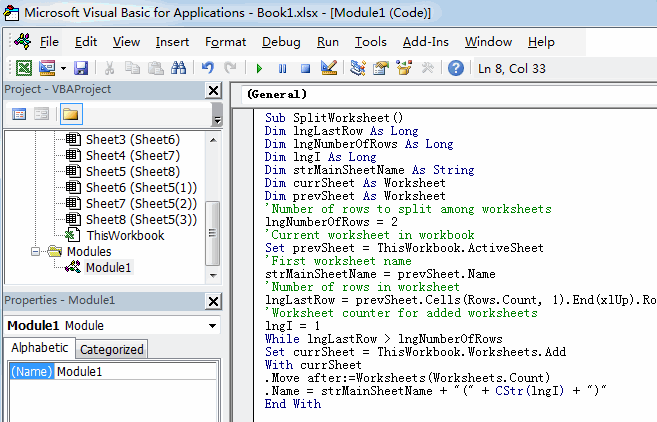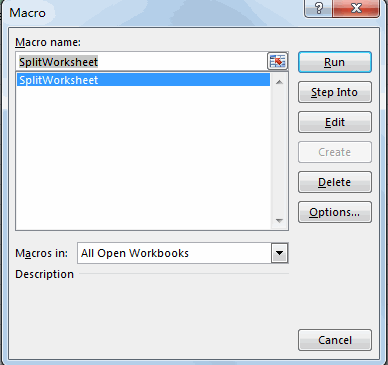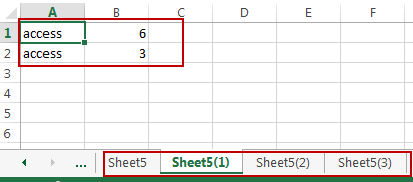# How to Split Data into Multiple Worksheets with Set Number of Rows (VBA) in Excel

This post will guide you how to split data into multiple worksheets based on row count in Excel. How do I split data into multiple worksheets by row count with VBA Macro in Excel 2010/2013/2016.

## Split Data into Multiple Worksheets by Row Count

Assuming that you have a list of data with 10 rows in your worksheet, and you want to split data into multiple worksheets by row count of every 2 of data. How to do it. You can use an Excel VBA macro to achieve the result quickly. Just do the following steps:

#1 open your excel workbook and then click on “Visual Basic” command under DEVELOPER Tab, or just press “ALT+F11” shortcut.#2 then the “Visual Basic Editor” window will appear.

#3 click “Insert” ->”Module” to create a new module.#4 paste the below VBA code(get code from here) into the code window. Then clicking “Save” button.```Sub SplitWorksheet()
Dim lngLastRow As Long
Dim lngNumberOfRows As Long
Dim lngI As Long
Dim strMainSheetName As String
Dim currSheet As Worksheet
Dim prevSheet As Worksheet
'Number of rows to split among worksheets
lngNumberOfRows = 2
'Current worksheet in workbook
Set prevSheet = ThisWorkbook.ActiveSheet
'First worksheet name
strMainSheetName = prevSheet.Name
'Number of rows in worksheet
lngLastRow = prevSheet.Cells(Rows.Count, 1).End(xlUp).Row
lngI = 1
While lngLastRow > lngNumberOfRows
With currSheet
.Move after:=Worksheets(Worksheets.Count)
.Name = strMainSheetName + "(" + CStr(lngI) + ")"
End With

With prevSheet.Rows(lngNumberOfRows + 1 & ":" & lngLastRow).EntireRow
.Cut currSheet.Range("A1")
End With

lngLastRow = currSheet.Cells(Rows.Count, 1).End(xlUp).Row
Set prevSheet = currSheet
lngI = lngI + 1
Wend
End Sub```

#5 back to the current worksheet, then run the above excel macro. Click Run button.